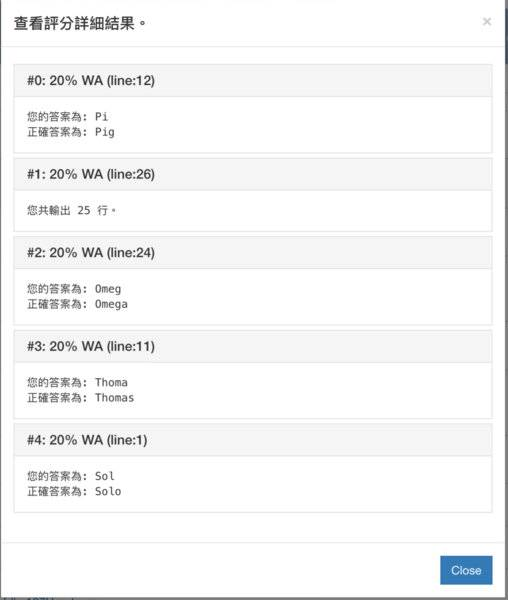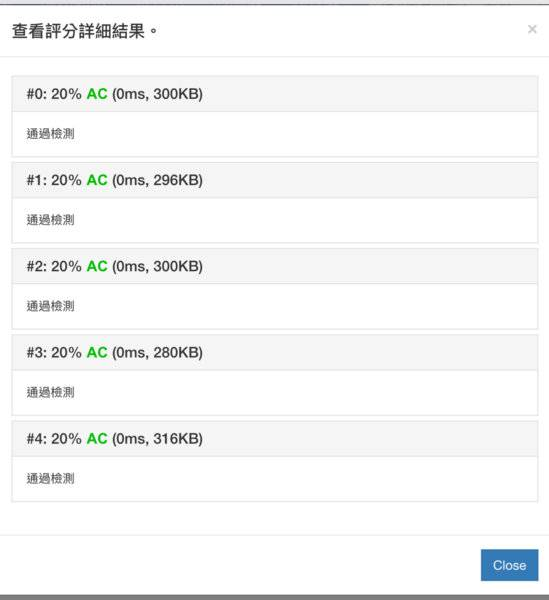# Why am I getting an extra blank line at the end of my output?

Note: this is not a homework question!!
The problem that this program should solve is turn a input like this:(no space and the end of the input string)
Code:
james shawn charles nicholas
into something like this:
Code:
James
Shawn
Charles
Nicholas
But I keep getting an excess line at the bottom of all outputs like this:
Code:
James
Shawn
Charles
Nicholas
<-This line
So my code send an excess ‘\n’,but I can’t find which part of my code went wrong.
My code:
Code:
#include<iostream>
#include<string.h>
using namespace std;
char n;
int lcv = 0;
int main(){
cin.getline(n,300);
cout<<char(toupper(n));
for(unsigned short lcv = 1;lcv<strlen(n)-1;lcv++){
while(n[lcv] != ' '){
if(lcv>=strlen(n))
break;
cout<<n[lcv];
lcv++;
}
cout<<'\n'<<char(toupper(n[lcv+1]));
lcv++;
}
return 0;
}

Last edited:

Stephen Tashi
The "break" statement only takes you out of the "while { ...} " code. It doesn't cause the program to skip the
Code:
cout<<'\n'<<char(toupper(n[lcv+1]));
statement.

•QuantumQuest and YoungPhysicist
Mark44
Mentor
To elaborate on what @Stephen Tashi said, break exits the innermost enclosing loop, which in this case is the while loop. It doesn't get you out of the outer loop.

1. Why have you declared lcv twice? You have a global variable of type int with this name, and also a variable of the same name of a different type that is local to the for loop. Having global variables like this is generally a bad practice.
2. Your variable names are not well chosen -- n is not a good name for a string variable. Single-letter names for variables are usually not good practice, except for loop control variables, which typically are called i, j, and the like. All other variable names should be chosen so that a reader can easily tell what they're supposed to represent.

•QuantumQuest and YoungPhysicist
jedishrfu
Mentor
If you are reading your data from a text file then the new line \n is likely at the end of your line.

Try nulling out the last character of your line of data and see if it goes away or subtract one from the string length.

Also be aware that on windows systems text lines will have a CRLF ie \r\n termination.

•QuantumQuest
verty
Homework Helper
A problem I see with your code is you are only checking for space characters, so you will print the \n character at the end of the line as though it were part of the last word which it isn't. For the cleanest code, you need a better check there. I personally would check for " \t\r\n" which should be good enough.

•QuantumQuest and jedishrfu
Thanks everyone,problem solved.I simply add another expression to jump out of two loops at once.Code:
#include<iostream>
#include<string.h>
using namespace std;
char n;
int main(){
cin.getline(n,300);
cout<<char(toupper(n));
for(unsigned short i = 1;i<strlen(n)-1;i++){
while(n[i] != ' '){
if(i>=strlen(n))
break;
cout<<n[i];
i++;
}
if(i>=strlen(n))                       // HERE
break;                                   // HERE
cout<<'\n'<<char(toupper(n[i+1]));
i++;
}
return 0;
}

pbuk
Gold Member
That is horrible. Try
Code:
#include<iostream>
#include<string.h>
using namespace std;
char input;
int main(){
cin.getline(n,300);
bool newword = true; /* If bool is not available (C < C99) use an int */
for (int i = 0; i < strlen(n) - 1; i++) {
if (n[i] == ' ') {
newword = 1;
} else if (newword) {
newword = false;
cout<<'\n'<<char(toupper(n[i]));
} else {
cout<<n[i];
}
}
return 0;
}

That is horrible. Try
Code:
#include<iostream>
#include<string.h>
using namespace std;
char input;
int main(){
cin.getline(n,300);
bool newword = true; /* If bool is not available (C < C99) use an int */
for (int i = 0; i < strlen(n) - 1; i++) {
if (n[i] == ' ') {
newword = 1;
} else if (newword) {
newword = false;
cout<<'\n'<<char(toupper(n[i]));
} else {
cout<<n[i];
}
}
return 0;
}
Thanks,but first,you forgot to replace my “n” with your “input”,second,it doesn’t work.It can’t get the last alphabet of every name.#### Attachments

pbuk
Gold Member
Sorry, didn't have a C compiler handy to test.
Code:
#include <iostream>
#include <string.h>

int main(){
// Get the input string.
char input;
std::cin.getline(input, 1000);

// Set a flag for the first word (if bool is not available (C < C99) use an int).
bool newword = true;
for (int i = 0; i < strlen(input); i++) {
// A space marks a new word but do not print it.
if (input[i] == ' ') {
newword = true;
// Print the current character, capitalizing if necessary.
} else if (newword) {
// Reset the flag for the next character.
newword = false;
std::cout << '\n' << char(toupper(input[i]));
} else {
std::cout << input[i];
}
}
return 0;
}

jtbell
Mentor
C++ has had the std::string data type for 20 years (probably since before YoungPhysicist was born). Which C++ textbook/resource is he/she using, that still uses C-strings (char arrays)?

C:
#include <iostream>
#include <string>

int main ()
{
std::cout << "Enter a list of words on a single line:" << std::endl;
std::string line;
std::getline (std::cin, line);

// Does not attempt to consolidate multiple spaces into a single newline.

for (int k = 0; k < line.size(); ++k)
{
if (line[k] == ' ')
std::cout << '\n';
else
std::cout << line[k];
}
std::cout << std::endl;
return 0;
}

Using the C++ 2011 standard, you can loop directly through the characters without using an index variable:

C:
#include <iostream>
#include <string>

int main ()
{
std::cout << "Enter a list of words on a single line:" << std::endl;
std::string line;
std::getline (std::cin, line);

// Does not attempt to consolidate multiple spaces into a single newline.
// Uses the range-based 'for' loop from C++ 2011 standard.

for (char thisChar : line)
{
if (thisChar == ' ')
std::cout << '\n';
else
std::cout << thisChar;
}
std::cout << std::endl;
return 0;
}

Oops, I forgot you want to capitalize the first letter of each word (name).

Last edited:
•YoungPhysicist, QuantumQuest and pbuk
C++ has had the std::string data type for 20 years (probably since before YoungPhysicist was born). Which C++ textbook/resource is he/she using, that still uses C-strings (char arrays)?

C:
#include <iostream>
#include <string>

int main ()
{
std::cout << "Enter a list of words on a single line:" << std::endl;
std::string line;
std::getline (std::cin, line);

// Does not attempt to consolidate multiple spaces into a single newline.

for (int k = 0; k < line.size(); ++k)
{
if (line[k] == ' ')
std::cout << '\n';
else
std::cout << line[k];
}
std::cout << std::endl;
return 0;
}

Using the C++ 2011 standard, you can loop directly through the characters without using an index variable:

C:
#include <iostream>
#include <string>

int main ()
{
std::cout << "Enter a list of words on a single line:" << std::endl;
std::string line;
std::getline (std::cin, line);

// Does not attempt to consolidate multiple spaces into a single newline.
// Uses the range-based 'for' loop from C++ 2011 standard.

for (char thisChar : line)
{
if (thisChar == ' ')
std::cout << '\n';
else
std::cout << thisChar;
}
std::cout << std::endl;
return 0;
}

Oops, I forgot you want to capitalize the first letter of each word (name).
Oops,I simply forgot it.(and all previous programs in my life)If you can connect to this forum’s servers via internet,try this one:
https://www.onlinegdb.com/
Now you got a C/C++ compiler handy!!!
And thanks @jtbell for pointing out the string problem,I have use an index variable for my past couple years,thank you for reminding me that!!!!

#### Attachments

pbuk
Gold Member
If you can connect to this forum’s servers via internet,try this one...
Thanks for the suggestion, although I currently use Repl.it.

EDIT: Mentor added code from repl.it for posterity:

C:
#include <iostream>
#include <string.h>

int main(){
// Get the input string.
char input;
std::cin.getline(input, 1000);

// Set a flag for the first word (if bool is not available (C < C99) use an int).
bool newword = true;
for (int i = 0; i < strlen(input); i++) {
// A space marks a new word but do not print it.
if (input[i] == ' ') {
newword = true;
// Print the current character, capitalizing if necessary.
} else if (newword) {
// Reset the flag for the next character.
newword = false;
std::cout << '\n' << char(toupper(input[i]));
} else {
std::cout << input[i];
}
}
return 0;
}

Last edited by a moderator:
Thanks for the suggestion, although I currently use Repl.it.
Great one!!

Svein
char *strtok(char *restrict s1, const char *restrict delimiters);
•